Circle

Chapter 11 Class 11 Conic Sections
Concept wise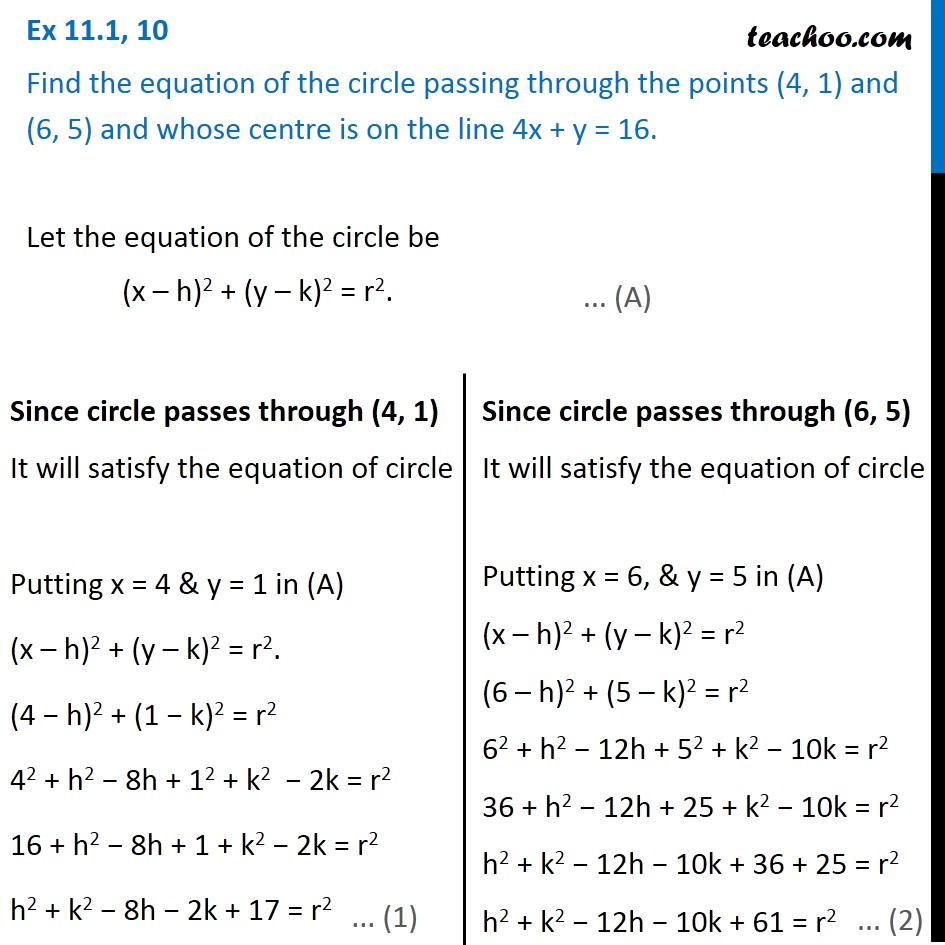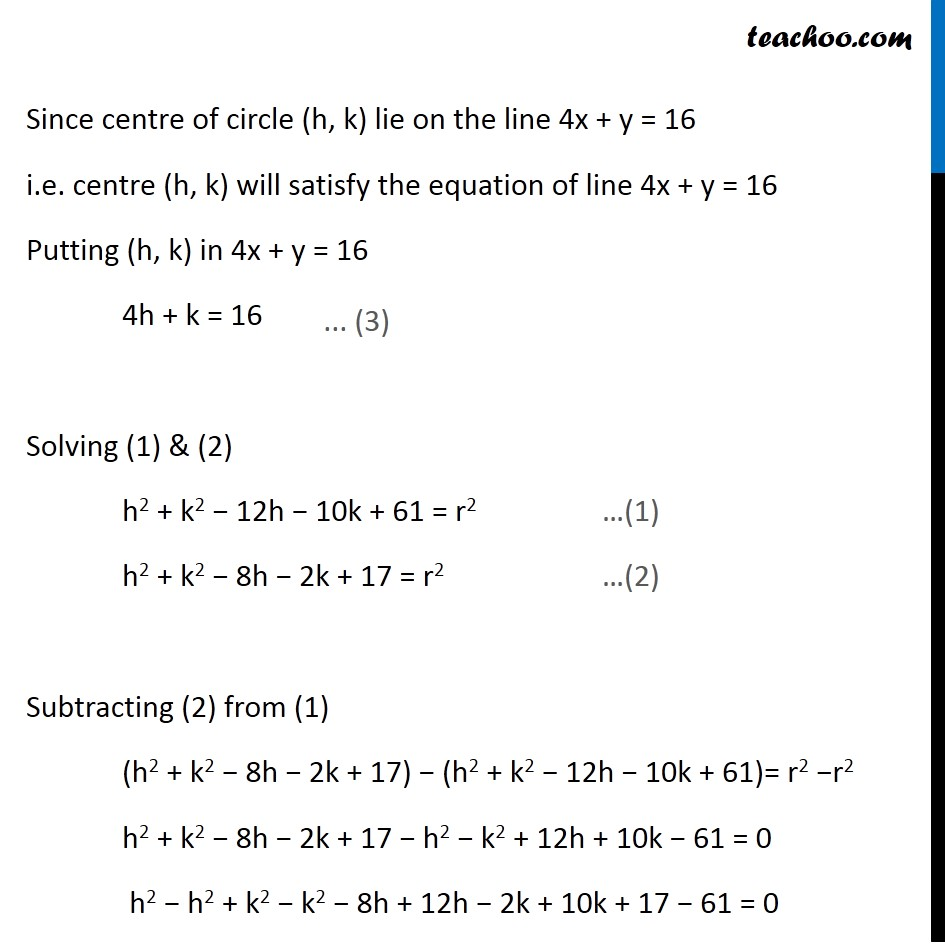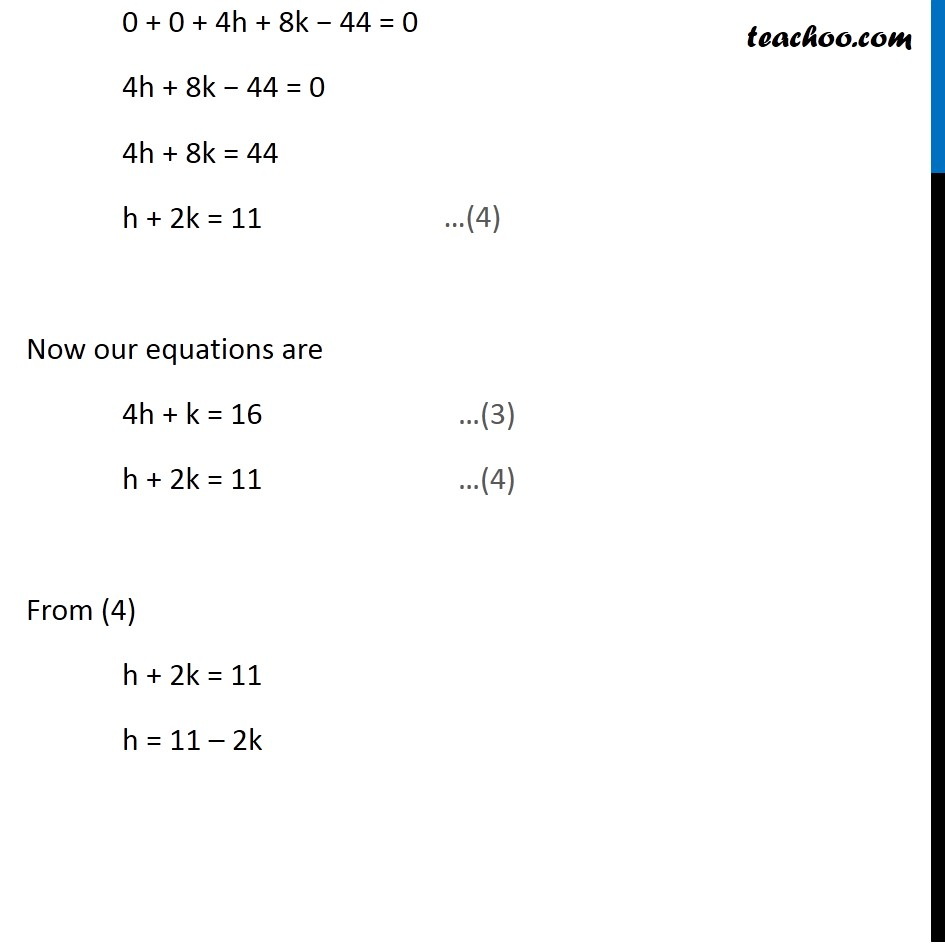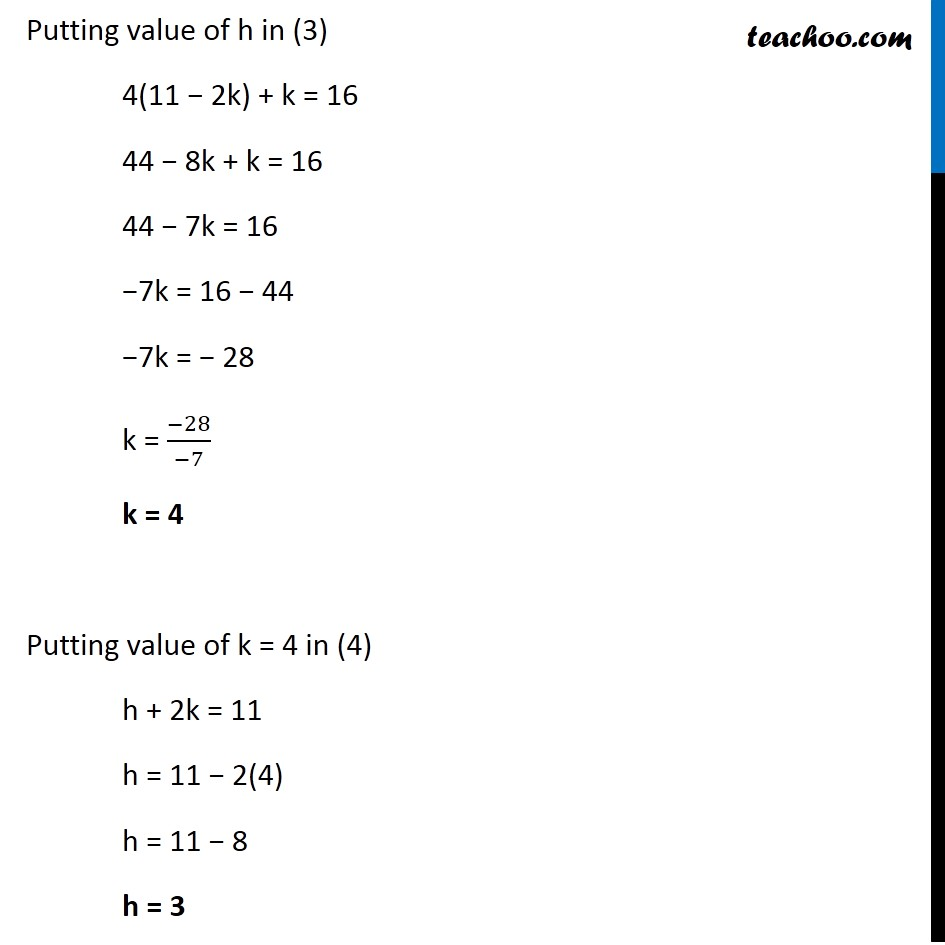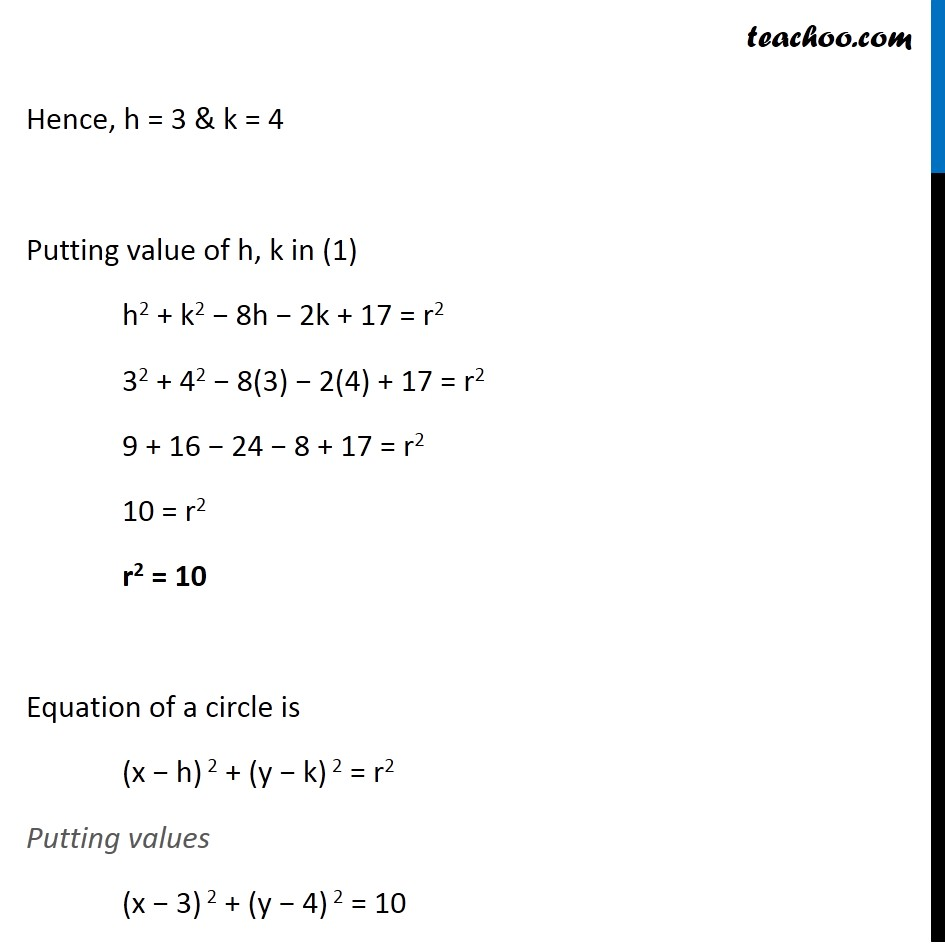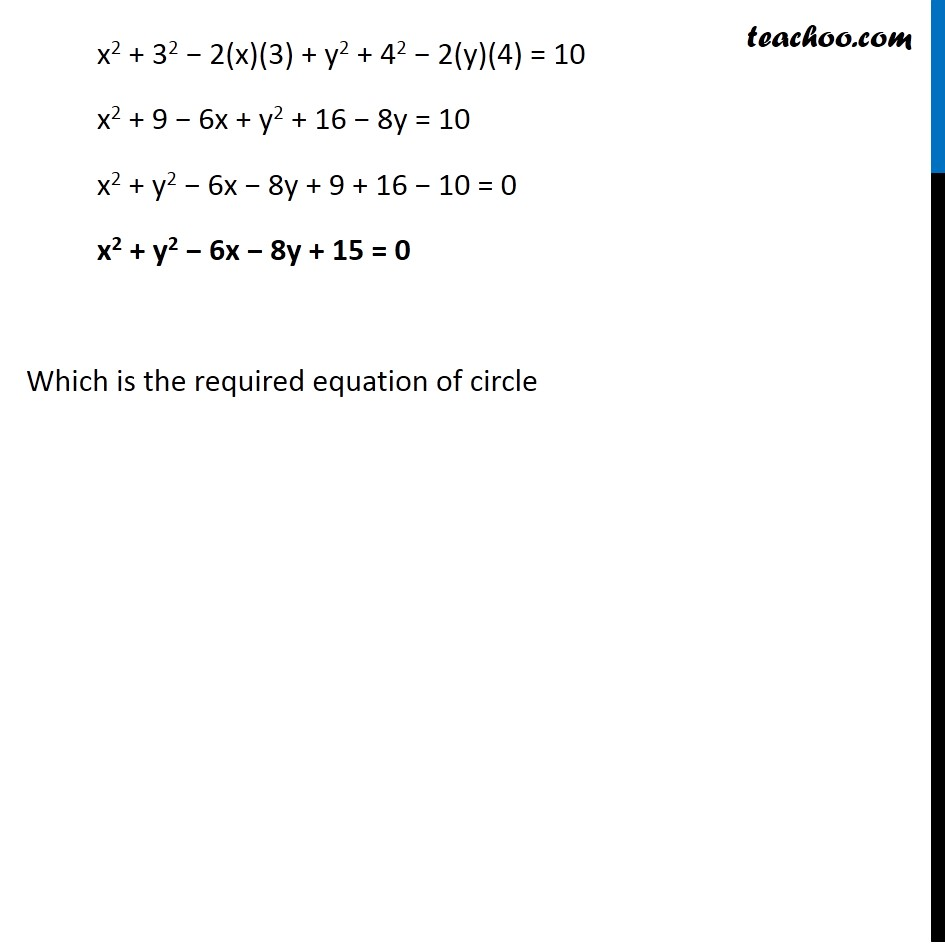Introducing your new favourite teacher - Teachoo Black, at only ₹83 per month

### Transcript

Ex 11.1, 10 Find the equation of the circle passing through the points (4, 1) and (6, 5) and whose centre is on the line 4x + y = 16. Let the equation of the circle be (x – h)2 + (y – k)2 = r2. Since circle passes through (4, 1) It will satisfy the equation of circle Putting x = 4 & y = 1 in (A) (x – h)2 + (y – k)2 = r2. (4 − h)2 + (1 − k)2 = r2 42 + h2 − 8h + 12 + k2 − 2k = r2 16 + h2 − 8h + 1 + k2 − 2k = r2 h2 + k2 − 8h − 2k + 17 = r2 Since circle passes through (6, 5) It will satisfy the equation of circle Putting x = 6, & y = 5 in (A) (x – h)2 + (y – k)2 = r2 (6 – h)2 + (5 – k)2 = r2 62 + h2 − 12h + 52 + k2 − 10k = r2 36 + h2 − 12h + 25 + k2 − 10k = r2 h2 + k2 − 12h − 10k + 36 + 25 = r2 h2 + k2 − 12h − 10k + 61 = r2 Since centre of circle (h, k) lie on the line 4x + y = 16 i.e. centre (h, k) will satisfy the equation of line 4x + y = 16 Putting (h, k) in 4x + y = 16 4h + k = 16 Solving (1) & (2) h2 + k2 − 12h − 10k + 61 = r2 …(1) h2 + k2 − 8h − 2k + 17 = r2 …(2) Subtracting (2) from (1) (h2 + k2 − 8h − 2k + 17) − (h2 + k2 − 12h − 10k + 61)= r2 −r2 h2 + k2 − 8h − 2k + 17 − h2 − k2 + 12h + 10k − 61 = 0 h2 − h2 + k2 − k2 − 8h + 12h − 2k + 10k + 17 − 61 = 0 0 + 0 + 4h + 8k − 44 = 0 4h + 8k − 44 = 0 4h + 8k = 44 h + 2k = 11 Now our equations are 4h + k = 16 …(3) h + 2k = 11 …(4) From (4) h + 2k = 11 h = 11 – 2k Putting value of h in (3) 4(11 − 2k) + k = 16 44 − 8k + k = 16 44 − 7k = 16 −7k = 16 − 44 −7k = − 28 k = (−28)/(−7) k = 4 Putting value of k = 4 in (4) h + 2k = 11 h = 11 − 2(4) h = 11 − 8 h = 3 Hence, h = 3 & k = 4 Putting value of h, k in (1) h2 + k2 − 8h − 2k + 17 = r2 32 + 42 − 8(3) − 2(4) + 17 = r2 9 + 16 − 24 − 8 + 17 = r2 10 = r2 r2 = 10 Equation of a circle is (x − h) 2 + (y − k) 2 = r2 Putting values (x − 3) 2 + (y − 4) 2 = 10 x2 + 32 − 2(x)(3) + y2 + 42 − 2(y)(4) = 10 x2 + 9 − 6x + y2 + 16 − 8y = 10 x2 + y2 − 6x − 8y + 9 + 16 − 10 = 0 x2 + y2 − 6x − 8y + 15 = 0 Which is the required equation of circle